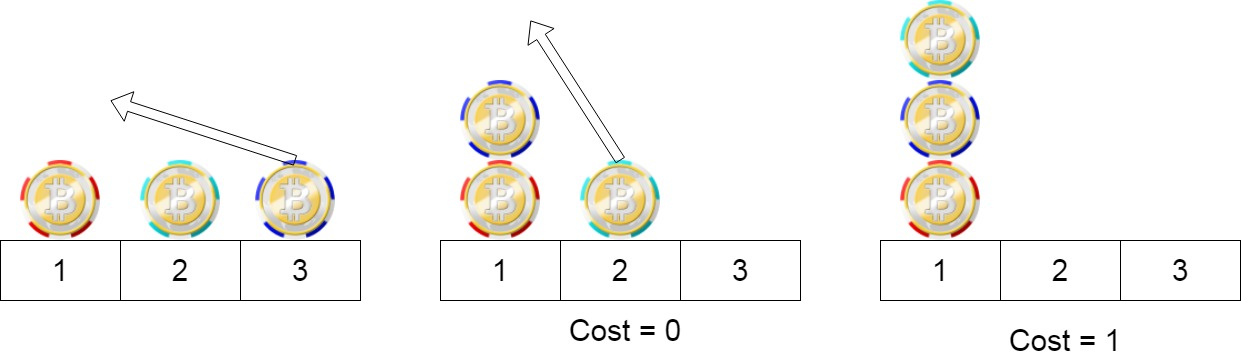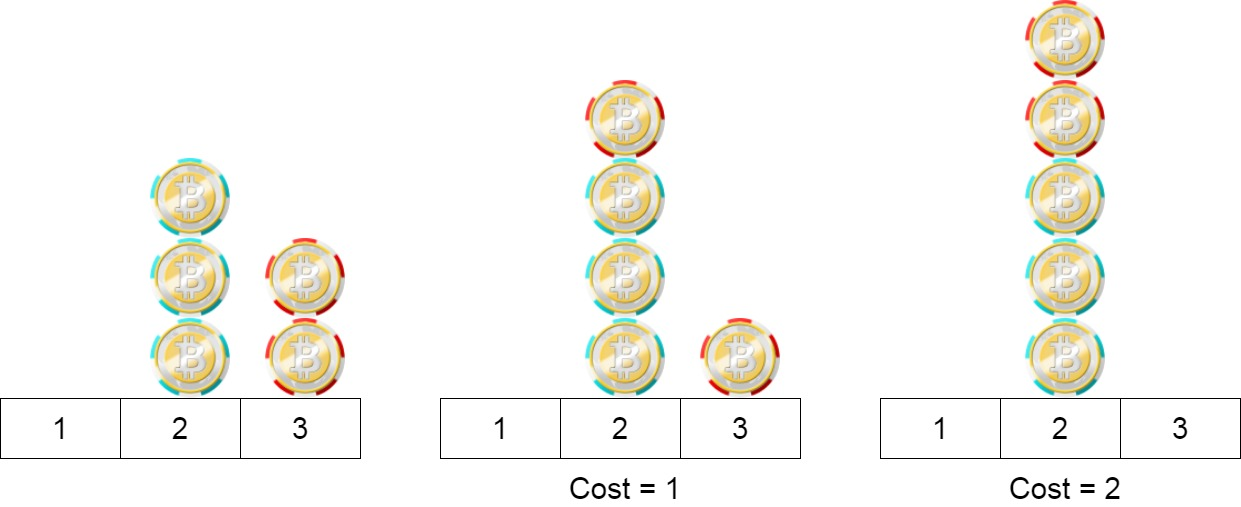##### Welcome to Subscribe On Youtube

Formatted question description: https://leetcode.ca/all/1217.html

# 1217. Minimum Cost to Move Chips to The Same Position (Easy)

We have n chips, where the position of the ith chip is position[i].

We need to move all the chips to the same position. In one step, we can change the position of the ith chip from position[i] to:

• position[i] + 2 or position[i] - 2 with cost = 0.
• position[i] + 1 or position[i] - 1 with cost = 1.

Return the minimum cost needed to move all the chips to the same position.

Example 1:Input: position = [1,2,3]
Output: 1
Explanation: First step: Move the chip at position 3 to position 1 with cost = 0.
Second step: Move the chip at position 2 to position 1 with cost = 1.
Total cost is 1.


Example 2:Input: position = [2,2,2,3,3]
Output: 2
Explanation: We can move the two chips at poistion 3 to position 2. Each move has cost = 1. The total cost = 2.


Example 3:

Input: position = [1,1000000000]
Output: 1


Constraints:

• 1 <= position.length <= 100
• 1 <= position[i] <= 10^9

Related Topics:
Array, Math, Greedy

## Solution 1.

• class Solution {
public int minCostToMoveChips(int[] chips) {
int evenCount = 0, oddCount = 0;
int length = chips.length;
for (int i = 0; i < length; i++) {
int chip = chips[i];
if (chip % 2 == 0)
evenCount++;
else
oddCount++;
}
return Math.min(evenCount, oddCount);
}
}

############

class Solution {
public int minCostToMoveChips(int[] position) {
int a = 0;
for (int p : position) {
a += p % 2;
}
int b = position.length - a;
return Math.min(a, b);
}
}

• // OJ: https://leetcode.com/problems/minimum-cost-to-move-chips-to-the-same-position/
// Time: O(N)
// Space: O(1)
class Solution {
public:
int minCostToMoveChips(vector<int>& A) {
int even = 0, odd = 0;
for (int n : A) {
if (n % 2) ++even;
else ++odd;
}
return min(even, odd);
}
};

• class Solution:
def minCostToMoveChips(self, position: List[int]) -> int:
a = sum(p % 2 for p in position)
b = len(position) - a
return min(a, b)

############

# 1217. Minimum Cost to Move Chips to The Same Position
# https://leetcode.com/problems/minimum-cost-to-move-chips-to-the-same-position/

class Solution:
def minCostToMoveChips(self, position: List[int]):
odd = even = 0

for p in position:
if p % 2 == 0: even += 1
else: odd += 1

return min(odd,even)

• func minCostToMoveChips(position []int) int {
a := 0
for _, p := range position {
a += p & 1
}
b := len(position) - a
if a < b {
return a
}
return b
}

• /**
* @param {number[]} position
* @return {number}
*/
var minCostToMoveChips = function (position) {
let a = 0;
for (let v of position) {
a += v % 2;
}
let b = position.length - a;
return Math.min(a, b);
};